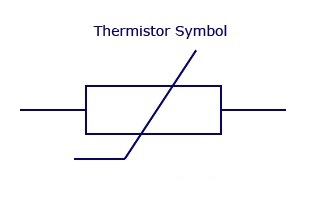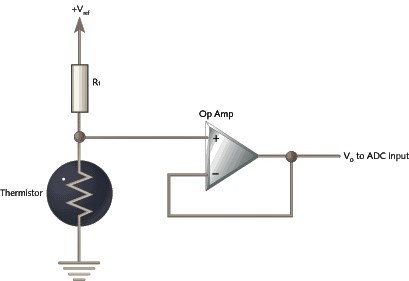# What are Thermistors and how they work?What are types of thermistor?

## What are thermistors?

A thermistor is one of the many temperature sensors used in temperature measurement. The thermistors are elements or semiconductors which varies their resistance when there is a change in temperature of the element.

Defenition: Thermistors are resistors whose resistance change rapidly with small change in temperature. In other words, when surrounding temperature changes, the current flow through the resistor changes such resistors are called as thermistors. The word thermistor is the combination of the words thermal and resistor.

Symbol for thermistor:## What are the types of thermistors?

There are mainly two types of thermistors, classified based on the resistance change. There are

• Positive Temperature Coefficient (PTC) Thermistor

• Negative Temperature Coefficient (NTC) Thermistor.

Positive Temperature Coefficient Thermistor:

PTC thermistors increase their resistance when there is an increase in temperature. These types of linear temperature sensors are mostly used in fuses for circuit protection.

Negative Temperature Coefficient Thermistor:

NTC thermistor decreases their resistance and increases conductivity when there is an increase in temperature. They are commonly semiconductors, when there is an increase in temperature free electrons are formed to increase the conductivity. They are used in temperature measuring devices.

## Circuit for Thermistor:Temperature change in thermistor changes the output voltage of the voltage follower output, where R1 resistance is constant.

Another kind of circuit commonly, shown in above figure, where the thermistor is connected to the base of the transistor. As the temperature increase base current increases then connect the collector and emitter and produce an output to the alarming system.

• Even for a small change in temperature, the resistance changes.

• Low cost

• Small in size

• Easy to dismantle